# How to use RIGHT function in Excel

In this article, we will learn about RIGHT function in Microsoft Excel.

RIGHT function is used to return the rightmost character or characters from a text string.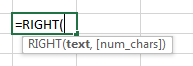With the help of examples, let us understand the use of RIGHT function. So, here we have dummy data with some sample text in column A.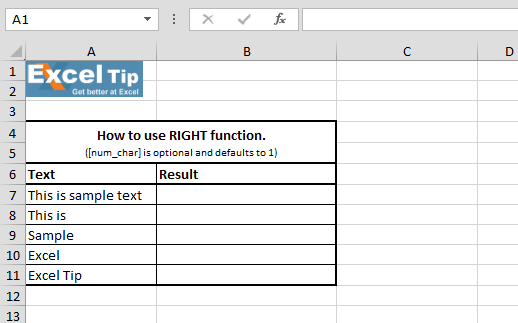1st Example:-

In this example, we have sentence in a cell from which we want to retrieve the text from the right in the cell.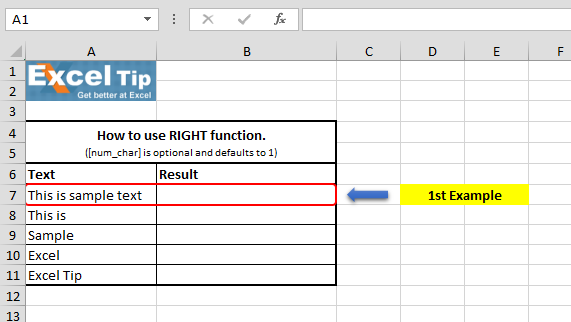Follow the steps given below:-

• Enter the function in cell B7
• =RIGHT(A7,4), Press Enter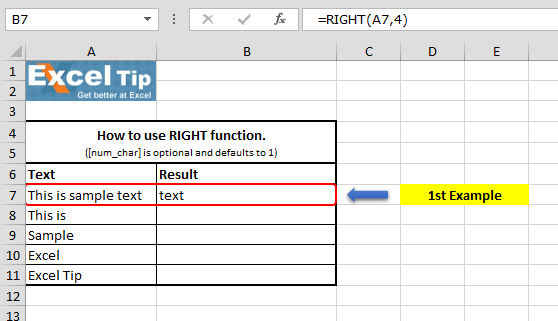Formula Explanation: In this function, first we have selected text from which we want to extract the text from the right side and as we want to extract 4 text, so we entered 4.

2nd Example:-

In this example, we will learn what happens when we do not enter number of characters?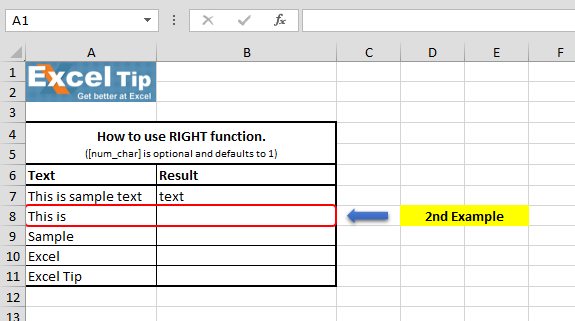Follow the steps given below:-

• Enter the function in cell B8
• =RIGHT(A8), Press Enter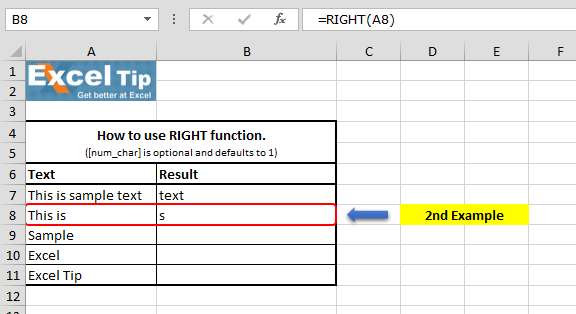The function has returned the rightmost character of that string, and in this case, it returns “s” which is the rightmost character of “This is” string.

3rd Example:-

In this example, we will see what happens when we enter 0 in 2nd argument (num_chars).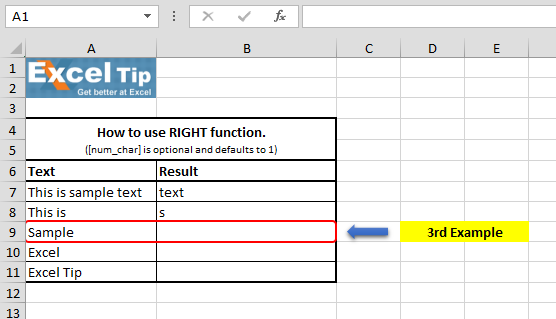Follow the steps given below:-

• Enter the function in cell B9
• =RIGHT(A9,0), Press Enter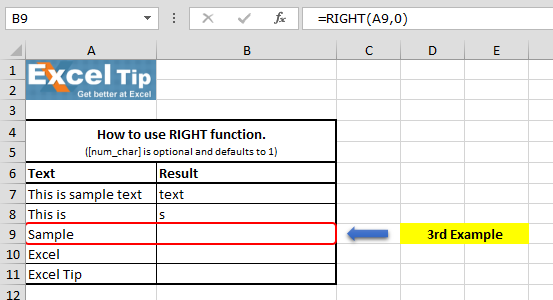The function has returned the empty cell because it is looking for “0” character to extract from this string and hence it returns blank cell.

4th Example:-

Now see what happens when we enter a negative value in number of character argument.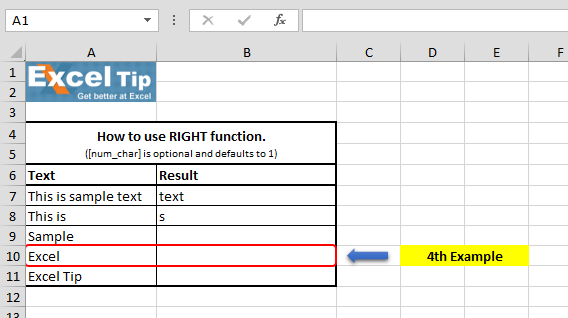Follow the steps given below:-

• Enter the function in cell B10
• =RIGHT(A10,-1), Press Enter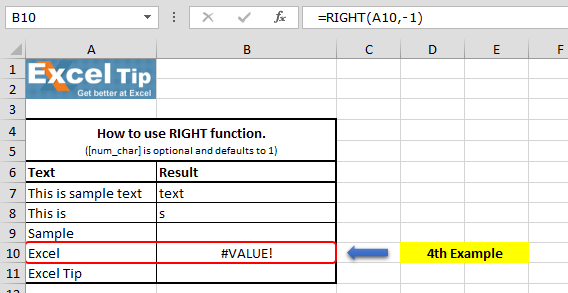It returns #VALUE error because RIGHT function does not allow any negative value.

5th Example:-

What happens in case we don’t know how many characters are to be extracted and we type any random number which is greater than the length of the original string?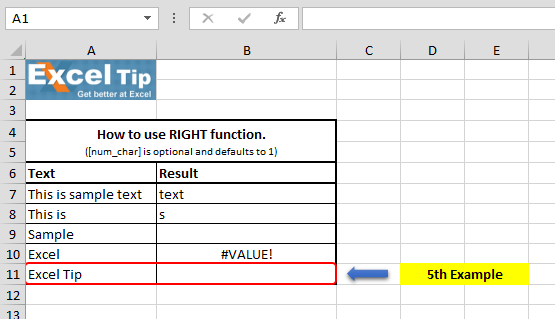Follow the steps given below:-

• Enter the function in cell B11
• =RIGHT(A11,15), Press Enter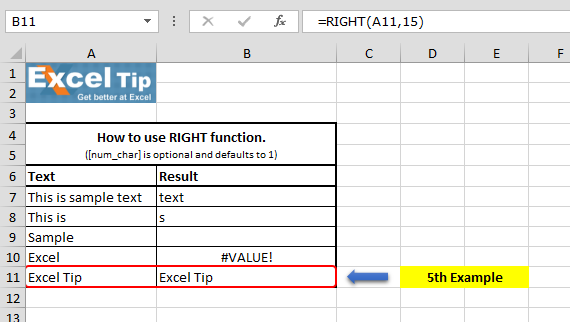It has extracted whole text from the cell, as you can see here we have typed 15 as the number of character whereas the length of this string is 9, which is less than the number of character we specified in the 2nd argument.

So this is how RIGHT function works in various ways in Excel.# Video: How to use RIGHT function in Microsoft Excel

Watch the steps in this short video, and the written instructions are above the video

If you liked our blogs, share it with your friends on Facebook. And also you can follow us on Twitter and Facebook.
We would love to hear from you, do let us know how we can improve, complement or innovate our work and make it better for you. Write us at info@exceltip.com

Related Articles:

Left Function in Microsoft Excel

Extract Text From A String In Excel Using Excel’s LEFT And RIGHT Function

Split Numbers and Text from String in Excel

Popular Articles:

50 Excel Shortcuts to Increase Your Productivity

How to use the VLOOKUP Function in Excel

How to use the COUNTIF function in Excel 2016

How to Use SUMIF Function in Excel

Terms and Conditions of use

The applications/code on this site are distributed as is and without warranties or liability. In no event shall the owner of the copyrights, or the authors of the applications/code be liable for any loss of profit, any problems or any damage resulting from the use or evaluation of the applications/code.